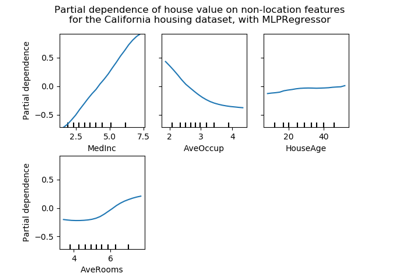# sklearn.inspection.partial_dependence¶

sklearn.inspection.partial_dependence(estimator, X, features, response_method='auto', percentiles=(0.05, 0.95), grid_resolution=100, method='auto')[source]

Partial dependence of features.

Partial dependence of a feature (or a set of features) corresponds to the average response of an estimator for each possible value of the feature.

Read more in the User Guide.

Parameters
estimatorBaseEstimator

A fitted estimator object implementing predict, predict_proba, or decision_function. Multioutput-multiclass classifiers are not supported.

X{array-like or dataframe} of shape (n_samples, n_features)

X is used both to generate a grid of values for the features, and to compute the averaged predictions when method is ‘brute’.

featuresarray-like of {int, str}

The feature (e.g. ) or pair of interacting features (e.g. [(0, 1)]) for which the partial dependency should be computed.

response_method‘auto’, ‘predict_proba’ or ‘decision_function’, optional (default=’auto’)

Specifies whether to use predict_proba or decision_function as the target response. For regressors this parameter is ignored and the response is always the output of predict. By default, predict_proba is tried first and we revert to decision_function if it doesn’t exist. If method is ‘recursion’, the response is always the output of decision_function.

percentilestuple of float, optional (default=(0.05, 0.95))

The lower and upper percentile used to create the extreme values for the grid. Must be in [0, 1].

grid_resolutionint, optional (default=100)

The number of equally spaced points on the grid, for each target feature.

methodstr, optional (default=’auto’)

The method used to calculate the averaged predictions:

Returns
averaged_predictionsndarray, shape (n_outputs, len(values), len(values), …)

The predictions for all the points in the grid, averaged over all samples in X (or over the training data if method is ‘recursion’). n_outputs corresponds to the number of classes in a multi-class setting, or to the number of tasks for multi-output regression. For classical regression and binary classification n_outputs==1. n_values_feature_j corresponds to the size values[j].

valuesseq of 1d ndarrays

The values with which the grid has been created. The generated grid is a cartesian product of the arrays in values. len(values) == len(features). The size of each array values[j] is either grid_resolution, or the number of unique values in X[:, j], whichever is smaller.

Warning

The ‘recursion’ method only works for gradient boosting estimators, and unlike the ‘brute’ method, it does not account for the init predictor of the boosting process. In practice this will produce the same values as ‘brute’ up to a constant offset in the target response, provided that init is a consant estimator (which is the default). However, as soon as init is not a constant estimator, the partial dependence values are incorrect for ‘recursion’. This is not relevant for HistGradientBoostingClassifier and HistGradientBoostingRegressor, which do not have an init parameter.

Examples

>>> X = [[0, 0, 2], [1, 0, 0]]
>>> y = [0, 1]

## Examples using sklearn.inspection.partial_dependence¶